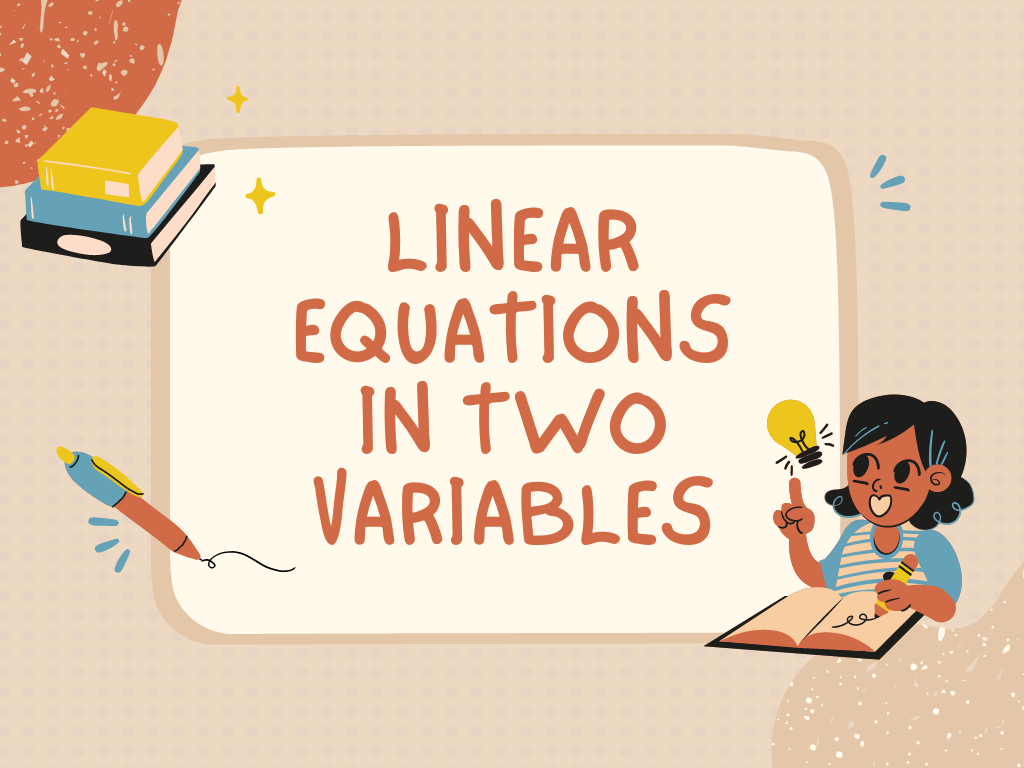## Linear Equations in Two Variables Class 9th

Standard Form of Linear Equations in Two Variables ax + by + c = 0 Example – Write each of the following equations in the form ax + by + c = 0 and indicates the values of a, b, and c in each case: (i) 3x + 4y = 7      (ii) x – 5 …